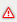Mathematics variables

The mathematics driver is used for defining formulas (linkage type of arithmetics, trigonometry, etc.) and calculating them by using the data from other process drivers. From the point of view of zenon, the Mathematics driver is a "normal" process driver. Variables are defined in the Editor (mathematics variable). The calculated values of the Mathematics variables are provided in the online operation. In order to define and use Mathematics variables, the Mathematics driver must be loaded first.

A formula must be defined for each mathematical variable of the Mathematics driver. Each formula must have at least one source variable. Any previously defined variable (even previously defined Mathematics variables) can be used as a source variable. The link is made with the signal resolution (technical values) of the variables.Attention You can configure a maximum of 4096 Mathematics variables with formulas. If you use more formulas, only the first 4096 will be executed during Runtime. Any further formulas will be ignored. You can sum up a maximum of 4096 numerical constants among all Mathematics formulas. Any further constants will be assumed as 0 during Runtime. If you require a larger number of variables/constants, we recommend the use of straton. Hint: straton is already included in zenon. In case straton is not included in your license, please contact the distributor that is responsible for you.